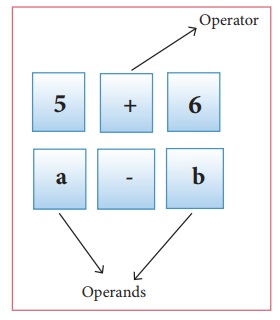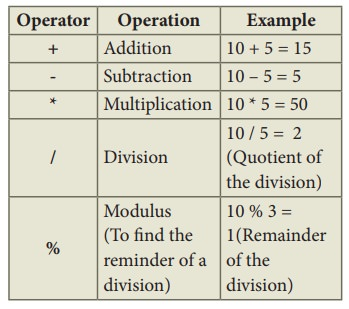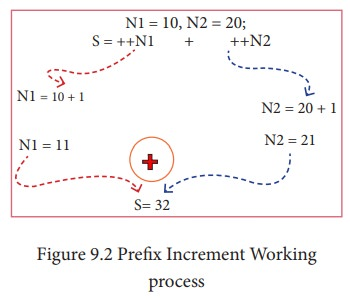Home | | Computer Science 11th std | Classification of C++ Operators

# Classification of C++ Operators

(1) Arithmetic Operators (2) Relational Operators (3) Logical Operators (4) Bitwise Operators (5) Assignment Operators (6) Conditional Operator (7) Other Operators

## Operators

The symbols which are used to do some mathematical or logical operations are called as “Operators”. The data items or values that the operators act upon are called as “Operands”.In C++, The operators are classified on the basis of the number of operands.

(i) Unary Operators - Require only one operand

(ii) Binary Operators - Require two operands

(iii) Ternary Operators - Require three operands

C++ Operators are classified as:

(1) Arithmetic Operators

(2) Relational Operators

(3) Logical Operators

(4) Bitwise Operators

(5) Assignment Operators

(6) Conditional Operator

(7) Other Operators

## (1) Arithmetic Operators

Arithmetic operators perform simple arithmetic operations like addition, subtraction, multiplication, division etc.,• The above mentioned arithmetic operators are binary operators which requires minimum of two operands.

### Increment and Decrement Operators

++ (Plus, Plus) Increment operator

-- (Minus, Minus) Decrement operator

An increment or decrement operator acts upon a single operand and returns a new value. Thus, these operators are unary operators. The increment operator adds 1 to its operand and the decrement operator subtracts 1 from its operand. For example,

• x++ is the same as x = x+1;

It adds 1 to the present value of x

• x-- is the same as to x = x–1;

It subtracts 1 from the present value of x

The ++ or -- operators can be placed either as prefix (before) or as postfix (after) to a variable. With the prefix version, C++ performs the increment / decrement before using the operand.For example:  N1=10, N2=20;

S = ++N1 + ++N2;

The following Figure explains the working process of the above statement.In the above example, the value of num is first incremented by 1, then the incremented value is assigned to the respective operand.

With the postfix version, C++ uses the value of the operand in evaluating the expression before incrementing / decrementing its present value.

For example: N1=10, N2=20;

S = N1++ + ++N2;

The following Figure explains the working process of the above statement.In the above example, the value assigned to operand N1 is taken into consideration, first and then the value will be incremented by 1.## (2) Relational Operators

Relational operators are used to determine the relationship between its operands. When the relational operators are applied on two operands, the result will be a Boolean value i.e 1 or 0 to represents True or False respectively. C++ provides six relational operators. They are,• In the above examples, the operand a is compared with b and depending on the relation, the result will be either 1 or 0. i.e., 1 for true, 0 for false.

• All six relational operators are binary operators.

## (3) Logical Operators

A logical operator is used to evaluate logical and relational expressions. The logical operators act upon the operands that are themselves called as logical expressions. C++ provides three logical operators.• AND, OR both are binary operators where as NOT is an unary operator.

Example: a = 5, b = 6, c = 7;## (4) Bitwise Operators

Bitwise operators work on each bit of data and perform bit-by-bit operation. In C++, there are three kinds of bitwise operators, which are:

(i) Logical bitwise operators

(ii) Bitwise shift operators

(iii) One’s compliment operators

### (i) Logical bitwise operators:

& Bitwise AND (Binary AND)

| Bitwise OR (Binary OR)

^ Bitwise Exclusive OR (Binary XOR)

• Bitwise AND (&) will return 1 (True)if both the operands are having the value 1 (True); Otherwise, it will return 0 (False)

• Bitwise OR (|) will return 1 (True) if any one of the operands is having a value 1 (True); It returns 0 (False) if both the operands are having the value 0 (False)

• Bitwise XOR (^) will return 1 (True) if only one of the operand is having a value 1 (True). If both are True or both are False, it will return 0 (False).

Truth table for bitwise operators (AND, OR, XOR)Example:

If a = 65, b=15

Equivalent binary values of 65 = 0100 0001; 15 = 0000 1111### (ii) The Bitwise shift operators:

There are two bitwise shift operators in C++, Shift left (<<) and Shift right (>>).

Shift left ( << )– The value of the left operand is moved to left by the number of bits specified by the right operand. Right operand should be an unsigned integer.

Shift right ( >> )– The value of the left operand is moved to right by the number of bits specified by the right operand. Right operand should be an unsigned integer.

### Example:

If a =15; Equivalent binary value of a is 0000 1111### (iii) The Bitwise one’s compliment operator:

The bitwise One’s compliment operator ~(Tilde),inverts all the bits in a binary pattern, that is, all 1’s become 0 and all 0’s become 1. This is an unary operator.

### Example:

If a =15; Equivalent binary values of a is 0000 1111## (5) Assignment Operator:

Assignment operator is used to assign a value to a variable which is on the left hand side of an assignment statement. = (equal to) is commonly used as the assignment operator in all computer programming languages. This operator copies the value at the right side of the operator to the left side variable. It is also a binary operator.C++ uses different types of assignment operators. They are called as Shorthand assignment operators.## (6) Conditional Operator:

In C++, there is only one conditional operator is used. ?: is a conditional Operator. This is a Ternary Operator. This operator is used as analternate to if … else control statement. We will learn more about this operator in later chapters along with if …. else structure.

## (7) Other Operators:### Precedence of Operators:

Operators are executed in the order of precedence. The operands and the operators are grouped in a specific logical way for evaluation. This logical grouping is called as an Association.

### The order of precedence:In C++, one or two operators may be used in different places with different meaning.

For example: Asterisk ( * ) is used for multiplication as well as for pointer to a variable.

Study Material, Lecturing Notes, Assignment, Reference, Wiki description explanation, brief detail
11th Computer Science : Chapter 9 : Introduction to C++ : Classification of C++ Operators |